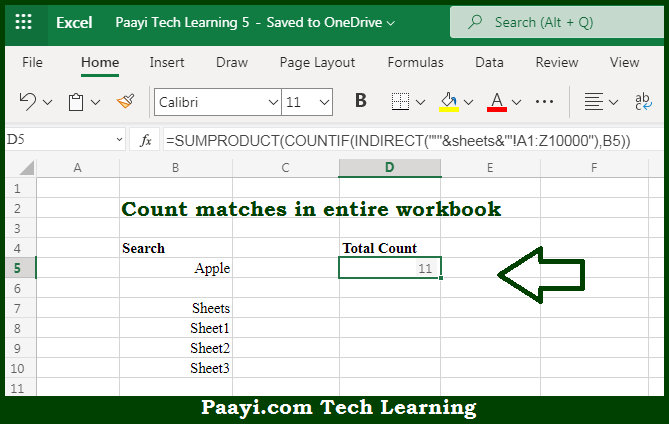# Learn How to Count Occurrences in Entire Workbook in Microsoft Excel

Written by | 0 Comments | 739 Views

In this article, you will learn how to COUNT various things in Microsoft Excel using a single or combination of functions and its purpose in Microsoft Excel. You will also get to know how to Count Occurrences in the Entire Workbook.

Count Occurrences in Entire Workbook in Microsoft Excel

The main purpose of this formula is to count matches in the entire workbook. Here we will learn how to count occurrences in the entire workbook in Microsoft Excel. That implies, with the help of a formula based on the SUMPRODUCT and COUNTIF functions you can able to count occurrences in the entire workbook. So, with the help of this formula, you can able to count matches in the entire workbook.

General Formula to Count Occurrences in Entire Workbook =SUMPRODUCT(COUNTIF(INDIRECT("'"&sheets&"'!"&rng),criteria))

The Explanation for the Count Occurrences in Entire WorkbookSo we know that with the help of the given formula above you can able to count matches in the entire workbook. Here we will learn how to count occurrences in the entire workbook in Microsoft Excel. As we know that, This workbook is having four worksheets. Three of the worksheets, "Sheet1", "Sheet2", and "Sheet3" contain 1000 random first names in a table. when we work from the inside out, we first have this expression inside the INDIRECT function. It should be noted that the range we are using in the formula, A1:Z10000, is arbitrary and should be adjusted to suit your data. So, with the help of this formula, you can able to count matches in the entire workbook.